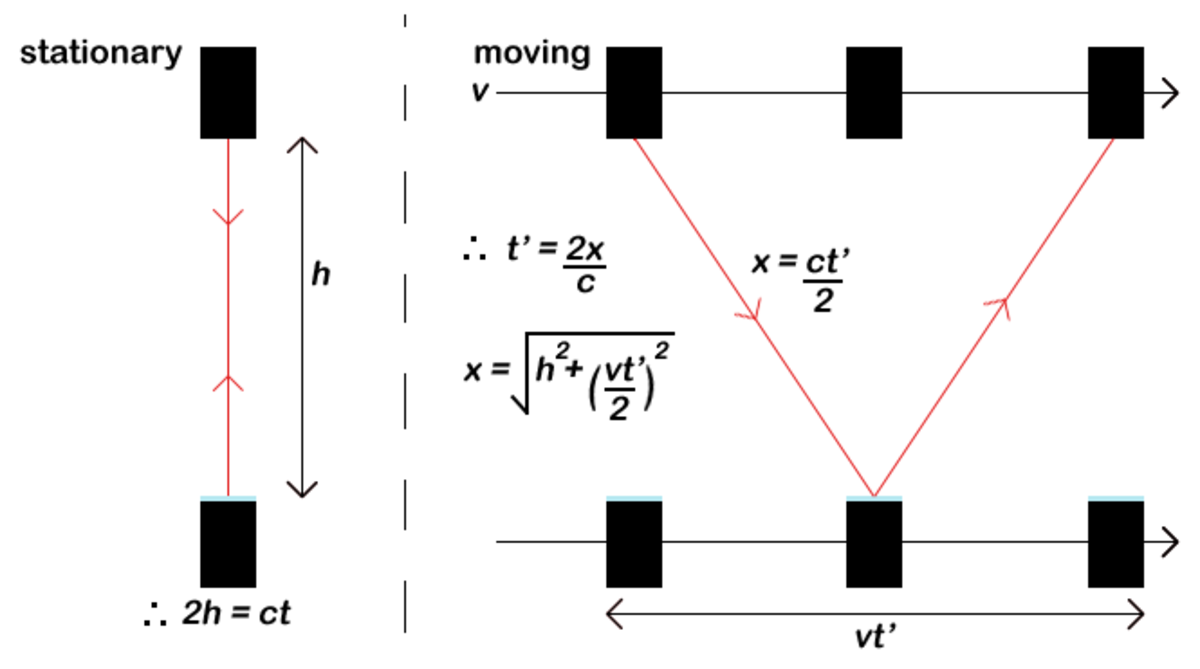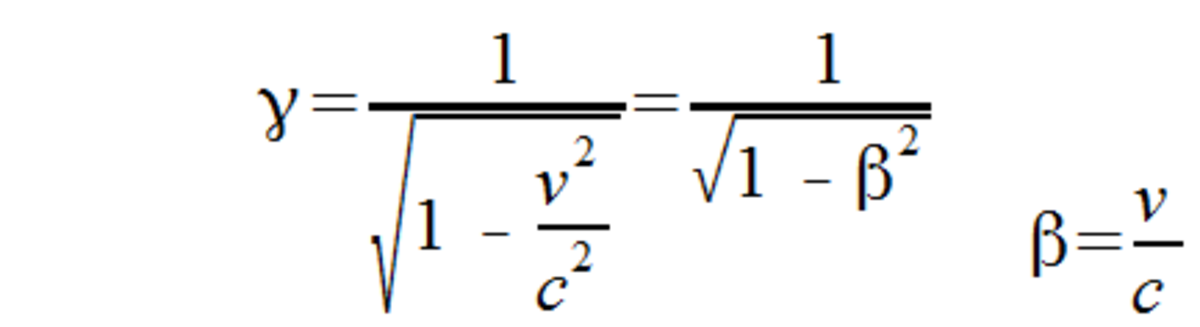# What Is Special Relativity?

Sam Brind holds a master's in physics with theoretical physics (MPhys) from the University of Manchester.

## Special Relativity

Special relativity is a very important physics theory introduced by Albert Einstein in 1905 (his 'miracle year'). At the time it completely revolutionised our understanding of space and time. The word relativity is well known and strongly associated with Einstein, but most people haven't actually studied the theory. Read on for a simple explanation of special relativity and its startling consequences.

## What Is a Frame of Reference?

To understand special relativity, the concept of a frame of reference needs to be understood. A frame of reference is a set of coordinates used to determine the positions and velocities of objects within that frame. Inertial frames of reference are a special case of frames that are moving at a constant speed. Special relativity exclusively deals with inertial frames of reference, hence the name special. Einstein's later theory of general relativity deals with the case of accelerating frames.

## Postulates

Einstein's theory of special relativity is based upon two postulates:

• The principle of relativity - The laws of physics are the same in all inertial frames of reference.

For example, an experiment performed within a train moving at a constant speed will produce the same results when performed on the train station platform. The train and the stationary platform are examples of different inertial frames of reference. Furthermore, if you were on this idealised train and couldn't see the outside then there is no way for you to determine that the train is moving.

• The principle of invariant light speed - The speed of light (in a vacuum), c, is the same in all inertial frames of reference.

This principle was the inspiration for Einstein's theory. Maxwell's theory of electricity and magnetism (1862) predicted a constant light speed but this was incompatible with classical Newtonian motion (1687). Einstein introduced special relativity to surpass Newtonian motion with a theory that was consistent with Maxwell's.

## A Light Clock

The light clock is a particularly simple example that can be used to demonstrate the consequences of special relativity upon time. The light clock is a theoretical clock that uses light to measure the time. Specifically, a pulse of light is reflected between two parallel mirrors that are spaced such that one second is the time for light to travel between the mirrors. The image below shows this setup as viewed by two different frames of reference. As viewed if the light clock is stationary relative to the observer, labelled as a stationary frame. The frame labelled as moving shows what an observer would see if the light clock is moving relative to the observer. Note that this is somewhat analogous to the aforementioned train example.The setup of our theoretical light clock in two different frames of reference. Notice how relative motion in the frame on the right modifies the observed path of light.

As shown by the simple maths in the above image (only Pythagoras theorem is required), the moving frame produces a longer path for the light to travel. However, due to the principle of invariant light speed, the light is travelling at the same speed in both frames. Hence, the time taken for the light pulse to reflect is longer in the moving frame, the associated second is longer and time runs slower. The exact formula for how much longer can easily be calculated and is given below.

## Time Dilation

Isn't the previous effect only valid for the special case of light clocks? If it was a special type of clock then you could compare a light clock to your normal wristwatch and determine if you were within a moving frame. This breaks the principle of relativity. Therefore, the effect must be equally true for all clocks.

The slowing down of time from relative movement is actually a fundamental property of our universe. In detail, observers will see time run slower in frames of reference that are moving relative to the observer's frame of reference. Or put simply, "moving clocks run slow". The formula for time dilation is given below and introduces the Lorentz factor.The lorentz factor, represented by the greek symbol gamma, is a common factor in the equations of special relativity.
Scroll to Continue

Due to the Lorentz factor, the effects of special relativity are only significant at speeds comparable to the speed of light. This is why we don't experience it's effects during our everyday experience. A good example of time dilation is muons incident on the atmosphere. A muon is a particle that can roughly be thought of as a "heavy electron". They are incident on the Earth's atmosphere as part of cosmic radiation and travel at near light speed. The average muon lifetime is only 2μs. Therefore, we wouldn't expect any muons to reach our detectors on earth. However, we do detect a significant amount of muons. From our frame of reference, the internal clock of the muon runs slower and hence the muon travels further due to special relativistic effects.

## Length Contraction

Special relativity also causes lengths to be changed by relative motion. Observers will see lengths shorten in frames of reference that are moving relative to the observer's frame of reference. Or put simply, "moving objects shrink along the direction of travel".

## Lorentz Transformation

To shift the coordinates of events between different inertial frames of reference the Lorentz transformation is used. The transform relations are given below alongside the geometry of the frames of reference.

## Relativity of Simultaneity

An important point to note, if you haven't already considered it, is the concept of simultaneous events. As the passage of time is relative to the frame of reference, simultaneous events will not be simultaneous in other frames of reference. It can be seen from the Lorentz transformation equations that simultaneous events will only remain simultaneous in other frames if they aren't spatially separated.

## Energy-Mass Equivalence

Ironically, Einstein's most famous equation actually drops out as a side effect of his theory of special relativity. Everything has a rest energy which is equal to the mass times the speed of light squared, energy and mass are in a sense equivalent. The rest energy is the minimum amount of energy a body can possess (when the body is stationary), motion and other effects can increase the total energy.

I will give two quick examples of this mass-energy equivalence. Nuclear weapons are the clearest example of converting mass into energy. Inside a nuclear bomb only a small mass of radioactive fuel is converted into a huge amount of energy. Conversely, energy can also be converted into mass. This is utilised by particle accelerators, such as the LHC, where particles are accelerated up to high energies and then collided. The collision can produce new particles with higher masses than the particles that were initially collided.